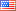# Revision history [back]

### How to polt ellipsoid with integraction?

I would like to plot ellipsoid $\dfrac{x^2}{a^2}+\dfrac{y^2}{b^2}+\dfrac{z^2}{c^2}=1$ in SageMath, where the parameters $a,b,c$ can be changed interactively. I found it what I want here https://www.geogebra.org/m/cqtAE6Sm. But I want it to do with Sage?

### How to polt ellipsoid with integraction?

I would like to plot ellipsoid $\dfrac{x^2}{a^2}+\dfrac{y^2}{b^2}+\dfrac{z^2}{c^2}=1$ in SageMath, where the parameters $a,b,c$ can be changed interactively. I found it what I want here https://www.geogebra.org/m/cqtAE6Sm. But I want it to do with Sage?

### How to polt ellipsoid with integraction?interaction?

I would like to plot ellipsoid $\dfrac{x^2}{a^2}+\dfrac{y^2}{b^2}+\dfrac{z^2}{c^2}=1$ in SageMath, where the parameters $a,b,c$ can be changed interactively. I found it what I want here https://www.geogebra.org/m/cqtAE6Sm. But I want it to do with Sage?the same in Sage. How can I do it?

### How to polt ellipsoid with interaction?

I would like to plot ellipsoid $\dfrac{x^2}{a^2}+\dfrac{y^2}{b^2}+\dfrac{z^2}{c^2}=1$ in SageMath, where the parameters $a,b,c$ can be changed interactively. I found it what I want here https://www.geogebra.org/m/cqtAE6Sm. But I want to do the same in Sage. How can I do it?5 NoneJohn Palmieri5718 ●18 ●51 ●126 http://www.math.washin...

### How to polt ellipsoid with interaction?interact?

I would like to plot ellipsoid $\dfrac{x^2}{a^2}+\dfrac{y^2}{b^2}+\dfrac{z^2}{c^2}=1$ in SageMath, where the parameters $a,b,c$ can be changed interactively. I found it what I want here https://www.geogebra.org/m/cqtAE6Sm. But I want to do the same in Sage. How can I do it?